##### Trigonometry For DummiesThe parent graph of cosine looks very similar to the sine function parent graph, but it has its own sparkling personality (like fraternal twins). Cosine graphs follow the same basic pattern and have the same basic shape as sine graphs; the difference lies in the location of the maximums and minimums. These extremes occur at different domains, or x values, 1/4 of a period away from each other. Thus, the two graphs are shifts of 1/4 of the period from each other.

Just as with the sine graph, you use the five key points of graphing trig functions to get the parent graph of the cosine function, f(x) = cos x. If necessary, you can refer to the unit circle for the cosine values to start with. As you work more with these functions, your dependence on the unit circle should decrease until eventually you don't need it at all. Here are the steps:

1. Find the values for domain and range.

Like with sine graphs, the domain of cosine is all real numbers, and its range is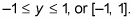2. Calculate the graph's x-intercepts.

Referring to the unit circle, find where the graph f(x)=cos x crosses the x-axis by finding the angles on the unit circle where the cosine is 0. You'll see that the graph f(x)=cos x crosses the x-axis twice —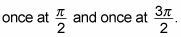Those crossings give you two coordinate points: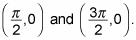3. Calculate the graph's maximum and minimum points.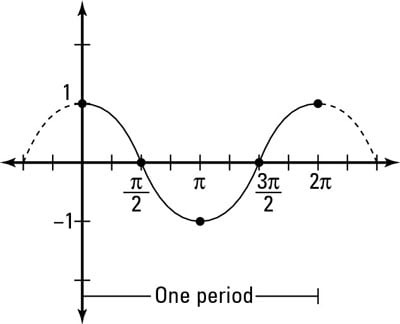Using your knowledge of the range for cosine from Step 1, you know the highest value that cos x can be is 1, which happens twice for cosine — once at an angle of 0 and once at an angle of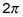(see the figure), giving you two maximums: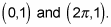The minimum value that cos x can be is –1, which occurs at an angle of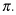You now have another coordinate pair at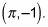4. Sketch the graph of the function.

The figure shows the full parent graph of cosine,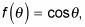with the five key points plotted.

The cosine parent graph has a couple of characteristics worth noting:

• It repeats every 2-pi radians. This repetition means it's a periodic function, so its waves rise and fall in the graph.

• It's symmetrical about the y-axis (in mathematical dialect, it's an even function). Unlike the sine function, which has 180-degree symmetry, cosine has y-axis symmetry. In other words, you can fold the graph in half at the y-axis and it matches exactly. The formal definition of an even function is f(x) = f(–x) — if you plug in the opposite input, you'll get the same output. For example,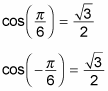Even though the input sign changed, the output sign for cosine stayed the same, and it always does for any theta value and its opposite.